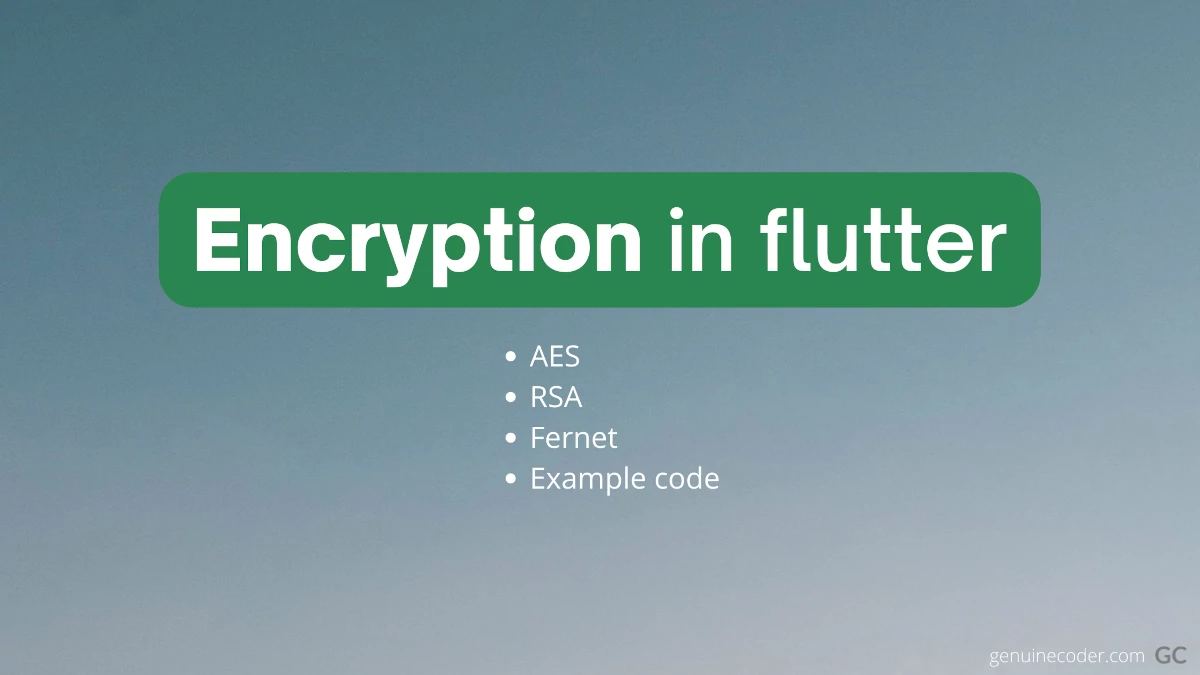Home Flutter Encryption and decryption in flutter

# Encryption and decryption in flutterPage Contents

This tutorial explores the data encryption and decryption using the dart language for the flutter apps. We come across the requirements of encrypting and decrypting data when we start building real-world applications.

For supporting encryption, we need to add a library called encrypt. It provides all the functionalities we require for encrypting, encoding encrypted data to base16 or base64, and of course for decryption.

Add the following line into your pubspec.yaml file under dependencies: section. This will add the ‘encrypt’ library into our project.

```encrypt: ^5.0.1
```

To use the library in the code, import it as follows.

```import 'package:encrypt/encrypt.dart';
```

## AES Encryption & Decryption

AES aka Advanced Encryption Standard is a very widely used symmetric block encryption technique. AES can use 128, 192 or 256 length keys.

#### How the encryption key bit length is calculated?

The AES-128 can have a 128-bit key. You can either use the Key.fromSecureRandom(16) function to generate 128-bit keys. Or else, you need to provide a 16 character string as a key.

For example,

• “I am 16-char key” contains 16 characters. Each character is 8 bit wide. It can be used as a 128-bit key.
• “This 32 char key have 256 bits!!” contains 32 characters. Each character is 8 bit wide. So, it will become 256-bit key.

#### Example program for AES-CBC encryption and decryption

Let’s understand the encryption and decryption with an example code. The following program utilizes AES-CBC-PKSC7 algorithm.

```import 'package:encrypt/encrypt.dart';

void main() {
final plainText = "Fallback! I repeat fallback to sector 12!!";
final key = "This 32 char key have 256 bits..";

print('Plain text for encryption: \$plainText');

//Encrypt
Encrypted encrypted = encryptWithAES(key, plainText);
String encryptedBase64 = encrypted.base64;
print('Encrypted data in base64 encoding: \$encryptedBase64');

//Decrypt
String decryptedText = decryptWithAES(key, encrypted);
print('Decrypted data: \$decryptedText');
}

///Accepts encrypted data and decrypt it. Returns plain text
String decryptWithAES(String key, Encrypted encryptedData) {
final cipherKey = Key.fromUtf8(key);
final encryptService = Encrypter(AES(cipherKey, mode: AESMode.cbc)); //Using AES CBC encryption
final initVector = IV.fromUtf8(key.substring(0, 16)); //Here the IV is generated from key. This is for example only. Use some other text or random data as IV for better security.

return encryptService.decrypt(encryptedData, iv: initVector);
}

///Encrypts the given plainText using the key. Returns encrypted data
Encrypted encryptWithAES(String key, String plainText) {
final cipherKey = Key.fromUtf8(key);
final encryptService = Encrypter(AES(cipherKey, mode: AESMode.cbc));
final initVector = IV.fromUtf8(key.substring(0, 16)); //Here the IV is generated from key. This is for example only. Use some other text or random data as IV for better security.

Encrypted encryptedData = encryptService.encrypt(plainText, iv: initVector);
return encryptedData;
}
```

The output of the program is as follows

```Plain text for encryption: Fallback! I repeat fallback to sector 12!!
Encrypted data in base64 encoding: WI18tczGAOQfiLKGyKyXKcuCZeK9d8K9ONUeVvPEnC8H86l0EBWa76drqdZpmcXO
Decrypted data: Fallback! I repeat fallback to sector 12!!
```

#### What is an initialization vector?

IV or initVector as denoted in the above code is known as initialization vector. It is an arbitrary data that can be used along with a secret key for more secure data encryption. This is needed because, multiple people can use the same key and create the same encrypted data which can then be compromised. Using IV along with key make sure that the probability of being someone else using IV and KEY is very less. In the above code snippet, the IV is derived from key. It is for example purpose only. Always use random generated IV.

## RSA Encryption and Decryption

RSA(Rivest Shamir Adleman) is a widely used public-key asymmetric encryption algorithm. You can do encryption and decryption with RSA in flutter with the encrypt library.

```import 'package:encrypt/encrypt.dart';
import 'package:pointycastle/asymmetric/api.dart';

void main() {

final plainText = 'Your plain text to be encrypted';
final encryptService = Encrypter(RSA(publicKey: publicKey, privateKey: privateKey));

//Encrypt
final encrypted = encryptService.encrypt(plainText);
final encryptedBase64 = encrypted.base64;
print('Encrypted data in base64 encoding: \$encryptedBase64');

//Decrypt
final decrypted = encryptService.decrypt(encrypted);
print('Decrypted data: \$decrypted');
}
```

You can find more elaborate example here in GitHub.

## More supported algorithms

The ‘encrypt’ library support some other encryption algorithms like Fernet and Salsa20.

## Conclusion

In this tutorial, we have explored the encryption and decryption support in dart for flutter apps. We have analyzed an example program for AES algorithm and went through an RSA algorithm example as well. If you liked this article, you might want to have a look into some other flutter articles I have written.

#### 1 COMMENT

1. […] In the above example, the IV is created from the key itself to keep the code easy to read. Use random data for IV for better security. Referred article for flutter encryption. […]